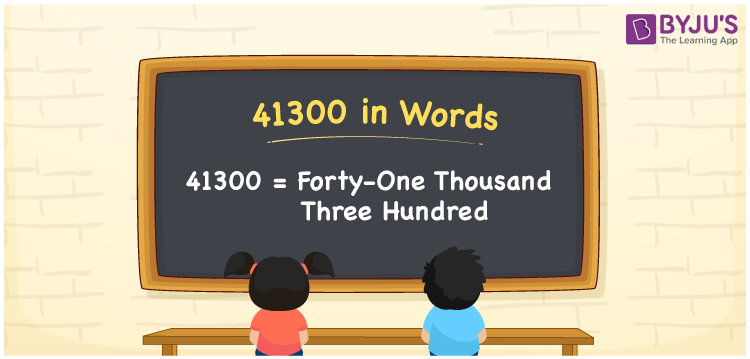# 41300 in Words

41300 in words is written as Forty-one thousand three hundred. In both the International System of Numerals and the Indian System of Numerals, 41300 is written as Forty-one thousand three hundred. The number 41300 is a Cardinal Number as it represents some quantity. For example, “that laptop costs 41300 rupees”, 41300 is used to quantify the price of the laptop.

 41300 in words Forty-one thousand three hundred Forty-one thousand three hundred in Number 41300

## 41300 in English Words

41300 in English words is read as “Forty-one thousand three hundred.”## How to Write 41300 in Words?

To write 41300 in words, we shall use the place value chart. In the place value chart, put 4 in the ten thousands, 1 in the thousands, 3 in the hundreds, and 0 in the rest. Let us make a place value chart to write the number 41300 in words.

 Ten Thousands Thousands Hundreds Tens Ones 4 1 3 0 0

Thus, we can write the expanded form as

4 × Ten Thousand + 1 × Thousand + 3 × Hundred + 0 × Ten + 0 × One

= 4 × 10000 + 1 × 1000 + 3 × 100 + 0 × 10 + 0 × 1

= 40000 + 1000 + 300 + 0 + 0

= 41300

= Forty-one thousand three hundred.

41300 is the natural number which is the successor of 41299 and predecessor of 41301.

41300 in words – Forty-one thousand three hundred

• Is 41300 an odd number? – No
• Is 41300 an even number? – Yes
• Is 41300 a perfect square number? – No
• Is 41300 a perfect cube number? – No
• Is 41300 a prime number? – No
• Is 41300 a composite number? – Yes

## Frequently Asked Questions on 41300 in Words

Q1

### How to write 41300 in words?

41300 in words is written as Forty-one thousand three hundred.
Q2

### How to write 41300 in words in the International and Indian System of Numerals?

In both, the system of numerals, 41300 in words, is written as Forty-one thousand three hundred.
Q3

### How to write 41300 in a place value chart?

In the place value chart, write 4 in the ten thousands, 1 in the thousands, 3 in the hundreds and 0 in the tens and the ones, respectively.